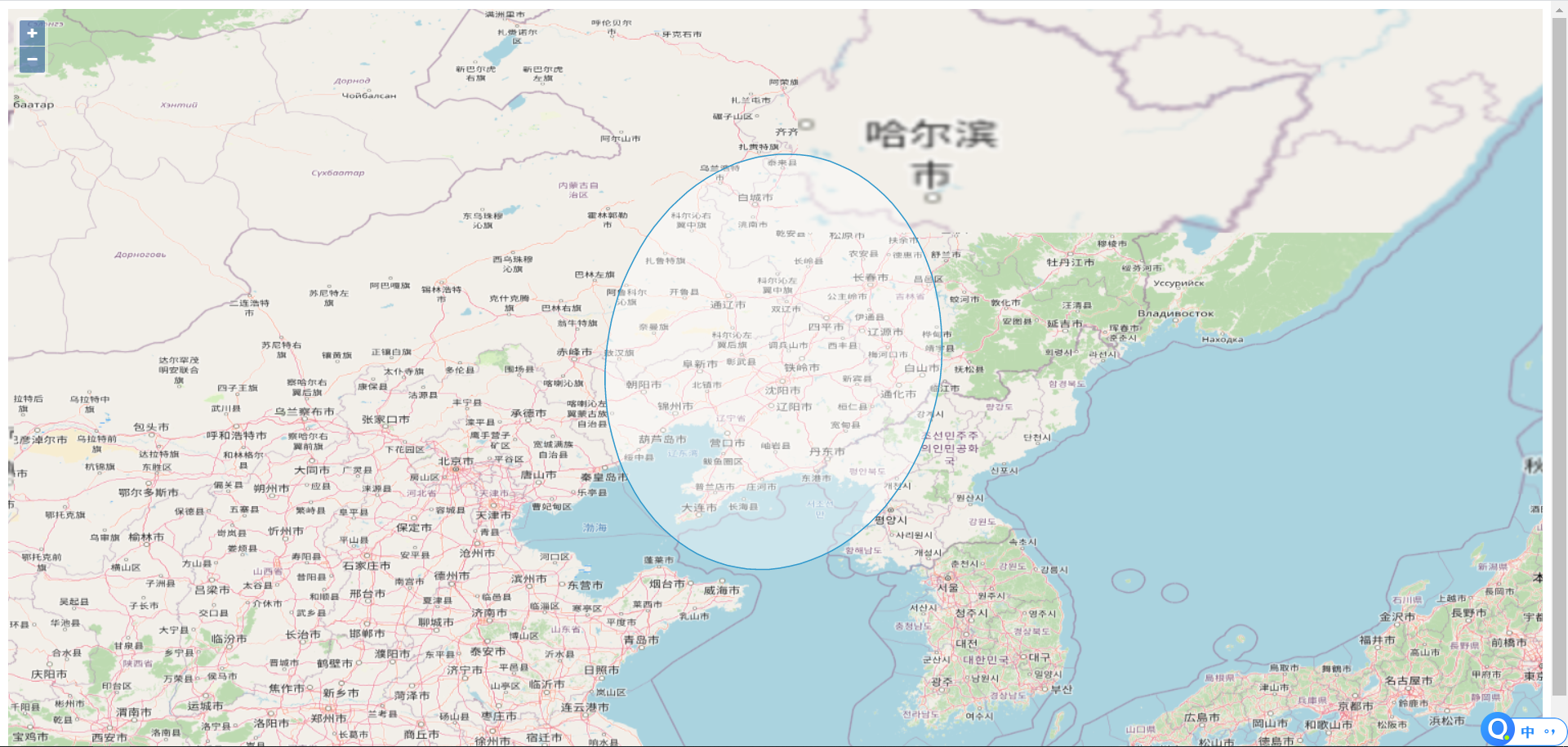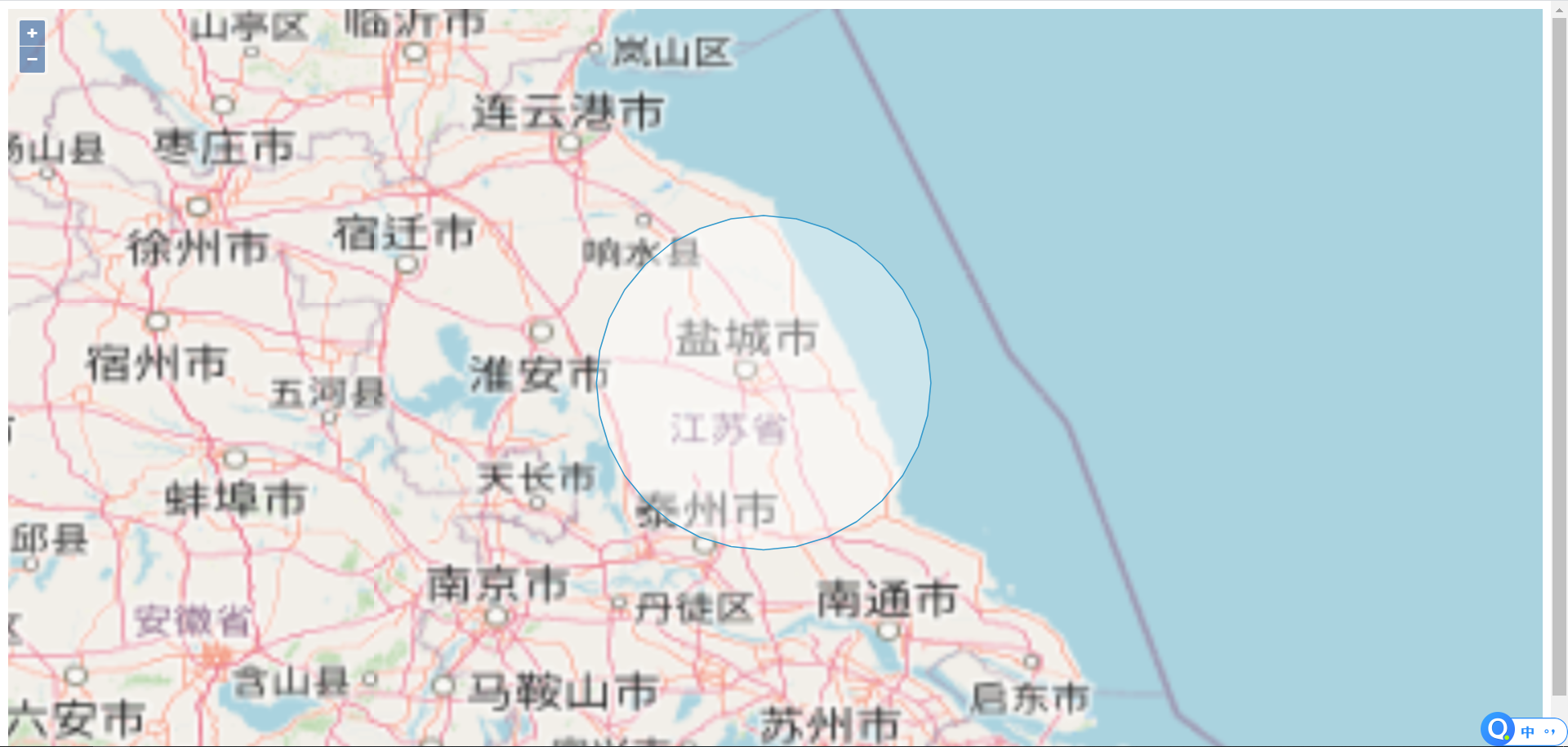# 写在前面

1、将长度（米）转换成经纬度的度数；
2、将圆形、椭圆、矩形、扇形用Java对象表示：用到com.vividsolutions.jts.util.GeometricShapeFactory工厂类和com.vividsolutions.jts.geom.Geometry对象；
3、判断线段与上述图形在地图上是否相交。

## 一、长度（米）转换为经纬度• 定义地球半径
``/** * 定义地球半径（米） */ private static final double R_EARTH = 6371000;``
• 定义地球赤道周长
`` /** * 定义地球赤道周长（米） */ private static final double P_EARTH = 2 * Math.PI * R_EARTH;``
• 将长度转换为纬度
`````` /** * 将Y轴的长度（米）转换成纬度 * @param length * @return */ public static double parseYLengthToDegree(double length){ //这种方式不对 半径会偏长 //将length长度转换为度数
// double yDegree = length / EARTH_RADIUS * 360;
// return yDegree; //使用Y轴做计量 会形成一个椭圆弧 这里在X轴也组处理暂时 没有找到方案 Double degree = ((2 * Math.PI * 3959 ) * 1609) / 360.0; Double dpmLat = 1 / degree; Double radiusLat = dpmLat * length; return radiusLat; }``````
• 将长度转换为经度
根据线段所在纬度，首先计算当前纬度的地球经线长，除以360得出每度经线的长度，即可将长度转换为经度。
`` /** * 根据所在纬度，将X轴的长度（米）转换成经度 * （因为不同纬度下，1°经度代表的长度不同） * @param y 所在纬度 * @param length 线段长度 * @return */ public static double parseXLengthToDegree(double y,double length){ //将角度（纬度）转换为弧度 double latRadian = Math.toRadians(y); //计算当前纬度地球周长 double latPEarth = P_EARTH * Math.cos(latRadian); //将length长度转换为度数 double xDegree = length / latPEarth * 360; return xDegree; }``

## 二、将圆形、椭圆、扇形用Geometry对象表示

• ### 表示圆形

``import com.vividsolutions.jts.geom.*;	import com.vividsolutions.jts.util.GeometricShapeFactory; /** * 根据圆形中心点经纬度、半径生成圆形（类圆形，32边多边形） * @param x 中心点经度 * @param y 中心点纬度 * @param radius 半径（米） * @return */ public static Polygon createCircle(double x, double y, final double radius) { //将半径转换为度数 double radiusDegree = parseYLengthToDegree(radius); //生成工厂类	private static GeometricShapeFactory shapeFactory = new GeometricShapeFactory();	//设置生成的类圆形边数 shapeFactory.setNumPoints(32); //设置圆形中心点经纬度 shapeFactory.setCentre(new Coordinate(x, y)); //设置圆形直径 shapeFactory.setSize(radiusDegree * 2); //使用工厂类生成圆形 Polygon circle = shapeFactory.createCircle(); return circle; }``

• ### 表示椭圆

`` /** * 根据中心点经纬度、长轴、短轴、角度生成椭圆 * @param x * @param y * @param macroaxis * @param brachyaxis * @param direction * @return */ public static Polygon createEllipse(double x,double y,double macroaxis,double brachyaxis,double direction){ //将长短轴转换为度数 double macroaxisDegree = parseYLengthToDegree(macroaxis); double brachyaxisDegree = parseYLengthToDegree(brachyaxis); //将夹角转换为弧度 double radians = Math.toRadians(direction); //设置中心点 shapeFactory.setCentre(new Coordinate(x,y)); //设置长轴长度 shapeFactory.setWidth(macroaxisDegree); //设置短轴长度 shapeFactory.setHeight(brachyaxisDegree); //设置长轴和X轴夹角 shapeFactory.setRotation(radians); //生成椭圆对象 Polygon ellipse = shapeFactory.createEllipse(); return ellipse; }``
• ### 表示扇形

`` /** * 根据中心点经纬度、半径、起止角度生成扇形 * @param x 经度 * @param y 纬度 * @param radius 半径（公里） * @param bAngle 起始角度（X轴正方向为0度，逆时针旋转） * @param eAngle 终止角度 * @param pointsNum 点数（往上参考可以给32） * @return */ public static Polygon createSector(double x,double y,double radius,double bAngle,double eAngle,int pointsNum){ //将半径转换为度数 double radiusDegree = parseYLengthToDegree(radius); //将起始角度转换为弧度 double bAngleRadian = Math.toRadians(bAngle); //将终止角度-起始角度计算扇形夹角 double angleRadian = Math.toRadians((eAngle - bAngle + 360) % 360); //设置点数 shapeFactory.setNumPoints(pointsNum); //设置中心点经纬度 shapeFactory.setCentre(new Coordinate(x, y)); //设置直径 shapeFactory.setSize(radiusDegree * 2); //传入起始角度和扇形夹角，生成扇形 Polygon sector = shapeFactory.createArcPolygon(bAngleRadian,angleRadian); return sector; }``

### 效果展示## 三、判断线段与图形是否相交

``````String lineStr = "LINESTRING(123.22 35.22,127.32 38.23)";
``````//判断线段是否包含在椭圆中